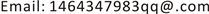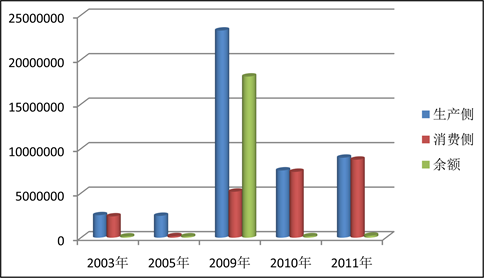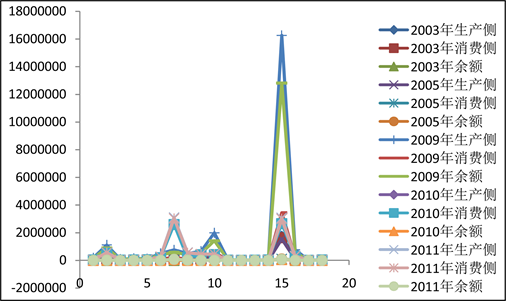﻿ 中国对日本贸易隐含碳排放余额的测算 China’s Measurement of Implied Carbon Emissions from Japan’s Trade

Statistics and Application
Vol.06 No.04(2017), Article ID:22287,6 pages
10.12677/SA.2017.64041

China’s Measurement of Implied Carbon Emissions from Japan’s Trade

Qun Wang

Jiangxi University of Finance and Economics, Nanchang JiangxiReceived: Sep. 19th, 2017; accepted: Oct. 5th, 2017; published: Oct. 12th, 2017ABSTRACT

Based on the input-output model, China’s carbon emissions in 2003, 2005, 2009, 2010 and 2011 were calculated from the aspects of production side, consumption side and balance carbon emissions. The results are as follows: On the whole, the hidden carbon in three aspects shows a rising trend, the balance shows a positive value, indicating that China is a net exporter for Japan. The fifth industry (electrical, natural gas, water supply industry), the seventh industry (coke, refined oil and nuclear fuel industry), the tenth industry (other non-metallic, mineral manufacturing) and the second industry (extractive industry), whose calculation of the value is relatively large, and some other industry values are relatively small.

Keywords:Input-Output, Production Side, Consumption Side, Implied CarbonCopyright © 2017 by author and Hans Publishers Inc.1. 引言及文献综述

2. 计算的方法与数据来源

1) 隐含碳的定义

2) 数据的来源以处理的说明

3) 基本的计算方法

$\left(\begin{array}{c}\begin{array}{c}{X}^{1}\\ {X}^{2}\end{array}\\ {X}^{3}\end{array}\right)=\left(\begin{array}{ccc}{A}_{11}& {A}_{12}& {A}_{13}\\ {A}_{21}& {A}_{22}& {A}_{23}\\ {A}_{31}& {A}_{32}& {A}_{33}\end{array}\right)\left(\begin{array}{c}\begin{array}{c}{X}^{1}\\ {X}^{2}\end{array}\\ {X}^{3}\end{array}\right)+\left(\begin{array}{c}{Y}_{11}+{\sum }_{i\ne 1}{Y}_{1i}\\ {Y}_{22}+{\sum }_{i\ne 2}{Y}_{2i}\\ {Y}_{33}+{\sum }_{i\ne 3}{Y}_{3i}\end{array}\right)\text{\hspace{0.17em}}\text{\hspace{0.17em}}\text{\hspace{0.17em}}\text{\hspace{0.17em}}\left(i=1,2,3\right)$

$X=AX+Y$ (1)

X表示各部门总产出列相量( $n×1$ )，A表示直接消耗系数( $n×n$ )，Y表示的是各部门最终使用的列相量。

WIOD投入产出数据库提供了41个欧盟国家、35个行业之间的经济往来以及行业之间的相互关系。世界投入产出表的形式和中国投入产出表的形式基本一致。都满足最基本的投入产出模型，当以中国为研究对象时。中国的总产出X可表示为  ：

$X={\left(I-{A}_{11}\right)}^{-1}\left[\left({A}_{12}{X}^{2}+{A}_{13}{X}^{3}\right)+{Y}_{11}+{Y}_{12}+{Y}_{13}\right]\text{\hspace{0.17em}}\text{\hspace{0.17em}}\text{\hspace{0.17em}}\text{\hspace{0.17em}}\left(i=2,3\right)$ (2)

$\text{I}={E}^{1}{B}_{11}{Y}_{11}+{E}^{1}{B}_{11}{A}_{12}{B}_{22}\left({Y}_{21}+{A}_{21}{B}_{11}{Y}_{11}\right)$ (3)

$Ⅱ={E}^{1}{B}_{11}\left({A}_{12}{X}^{2}+{Y}_{12}\right)-{E}^{1}{B}_{11}{A}_{12}{B}_{22}\left({Y}_{21}+{A}_{21}{B}_{11}{Y}_{11}\right)$ (4)

$Ⅲ={E}^{2}{B}_{22}{Y}_{21}+{E}^{2}{B}_{22}{A}_{21}{B}_{11}{Y}_{11}+{E}^{2}{B}_{22}{A}_{23}{B}_{33}\left({Y}_{31}+{A}_{31}{B}_{11}{Y}_{11}\right)$ (5)

3. 结果的分析

1) 整体分析Figure 1. China 2003, 2005, 2009, 2010, 2011, Japan, the three aspects of the overall distribution of hidden carbon (unit: million tons)

2) 从三大计算分析

3) 从18大行业分析Table 2. China 2003, 2005, 2009, 2010 2011 Production side, consumption side and trade balance implied carbon emissions (10,000 tons)Figure 2. China’s 2003, 2005, 2009, 2010, 2011, Japan’s 18 major industries, the implied carbon emissions

4. 结论与建议

1) 结论

a) 从整体来看，生产侧、消费侧及余额测算的隐含碳排放量大致呈现出上升的趋势，生产侧和消费侧方面计算的隐含碳排放平均增长速度是很大的，但是贸易隐含碳余额的平均增长速度相对较低的，甚至有些行业出现了负值。

b) 2003年、2005年、2009年、2010年及2011年这五年间中国的生产侧碳排放量总是大于中国消费侧碳排放量，致使贸易隐含碳排放的余额出现贸易顺差，及中国对于日本是净出口国。

c) 从18行业来看，第十五行业(电气、天然气、水供应业)、第七行业(焦炭、精炼石油及核燃料业)、第十行业(其他非金属、矿物制造业)及第二行业(采掘业)在中国对于日本的生产侧、消费侧及贸易隐含碳余额的测算中数值较大，其他一些行业数值相对较小。

2) 建议

a) 根据计算的结果，贸易隐含碳余额是正值，所以无论从哪些方面对隐含碳进行责任承担上都是存在争议的，比较好的方法就是将生产的和消费的产生的隐含碳都考虑进去，然后对净出口隐含碳进行责任分担，本文建议可以采取“谁受益谁承担”的角度进行分担。

b) 无论是从中国的生产侧还是消费侧的碳排放来看，数值都是相当的大，所以中国有必要去关注这个问题，考虑是什么原因导致的这种现状，比如：技术、设备及操作方法等。

c) 从18大行业来看，中国的某些行业的碳排放量是相当大的，而有些行业变化不大，这体现了中国的在某些行业上发展的不平衡，可以采取一些有效的措施去加强一些行业的发展。

China’s Measurement of Implied Carbon Emissions from Japan’s Trade[J]. 统计学与应用, 2017, 06(04): 367-372. http://dx.doi.org/10.12677/SA.2017.64041

1. 1. 陈楠, 刘学敏. 垂直专业化下中日贸易“隐含碳”实证研究[J]. 统计研究, 2016(3): 80-87.

2. 2. 向蓉美. 投入产出法[M]. 成都: 西南财经大学出版社, 2007.

3. 3. 王文治, 陆建明. 中国对外贸易隐含碳排放余额的测算与责任分担[J]. 统计研究, 2016(8): 12-19.

4. 4. 北京大学中国经济研究中心课题组. 中国出口贸易中的垂直专业化与中美贸易[J]. 世界经济, 2006(5): 3-11.

5. 5. 魏思超. 中国出口贸易隐含碳的测算及影响因素研究[D]: [硕士学位论文]. 长沙: 湖南大学, 2012.